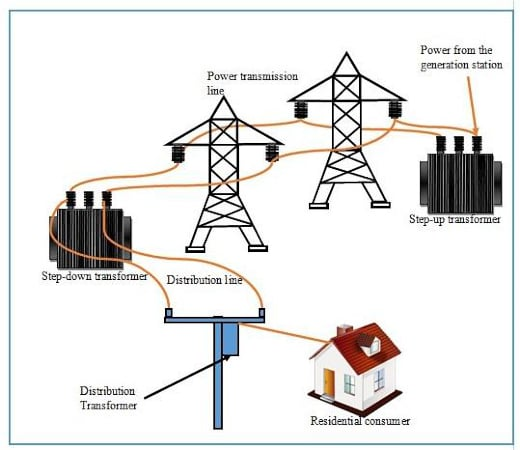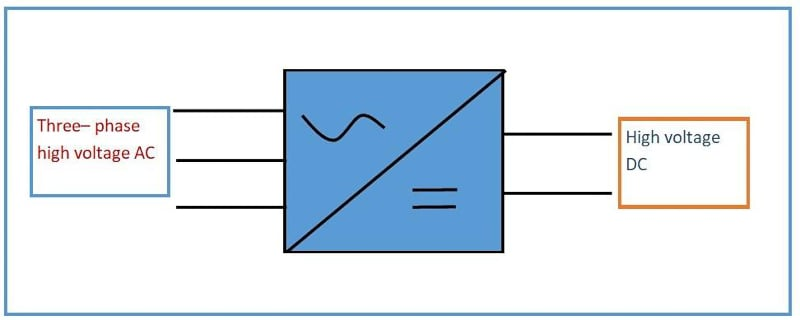Technical Article

# Understanding Advances in Transmission and Distribution

April 10, 2023 by Bob Odhiambo

## Here is an in-depth look at power transmission and distribution systems and the components that help optimize them, such as converters, machine learning and advanced analytics, load management, and distribution automation.

In a power grid system, transmission and distribution are crucial to ensuring that electricity is delivered efficiently and reliably. There have been notable improvements in power transmission and distribution technology over the years. This article will dive deeper into these innovations, including high-voltage direct current converters (HVDC), advanced analytics and machine learning algorithms, load management technology, and distribution automation.### High-voltage Direct Current Converters

HVDC converters are electrical devices that convert alternating current (AC) to direct current (DC) and vice versa, allowing for the interconnection and long-distance transmission of power into the grid system in different frequencies. The control algorithm and advances in digital technology in HVDC have resulted in a notable improvement in the reliability, efficiency, and flexibility of the system.##### Figure 2. High-voltage direct current converter symbol. Image used courtesy of Bob Odhiambo

Minimal losses in power transmission over long distances are one of the primary advantages of HVDC. This is made possible since the transmission of DC power can be done at higher voltages than AC power, thereby minimizing losses caused by increased resistance in the grid. The determination of the transfer capacity of power in the HVDC system is done using the maximum current and voltage that can be transmitted through the system. The power transfer capacity can be calculated using the following formula;

P = V×I

where P is the power in watts, V is the voltage in volts, and I is the current in amperes.

Example Calculation

An HVDC system can transmit a maximum voltage of 250 kV and a maximum current of 1000 A. Evaluate the maximum power transfer capacity in the system.

P = V×I

P = 250,000 volts×1000 amperes

P = 250,000,000 watts

P = 250 MW

The maximum power transfer function in the HVDC is therefore equal to 250 MW.

Another advantage of the technology in HVDC is the ability to integrate renewable energy sources into the grid system. Wind and solar can pose a challenge to their stability in power output. This is where HVDC come in to counteract this issue by smoothing out the variability of their output.

The performance and operation cost of the power transmitted can be affected by the efficiency of the HVDC converter. Losses in converters can be divided into AC losses resulting from filter circuit losses and transformer and DC losses resulting from the resistance of the power transmission line. To calculate the total converter losses, we sum up the AC and DC losses. Losses in AC can be evaluated using the following formula;

AC losses = Transformer losses + Filter circuit losses

For DC losses the formula below is used;

DC Losses = I2×R

Where R is the resistance in Ohms of the transmission line, and I is the current in amperes flowing through the line.

Example calculation

A DC transmission line has a resistance of 0.01 ohms. If the current flowing through it is 1000A, calculate the DC losses in the system.

Solution

DC Losses = 10002 × 0.01=10000 watts or 10 kW

If the transformer losses and the filter circuit losses in the system are 5000 watts and 3000 watts respectively, then the AC losses can be evaluated as follows:

AC losses = 5000+3000=8000 watts or 8 kW

The total converter loss can therefore be calculated as follows;

Total converter losses = 8 kW+10 kW=18 kW

By minimizing the losses, the efficiency of HVDC converters can be optimized to improve performance and lower operating costs.

### Advanced Analytics and Machine Learning

Advanced analytics and machine learning in the transmission and distribution of power in grid systems are becoming increasingly notable. The extraction of insights and data analysis using mathematical and statistical models is called advanced analytics. The extraction of insights and data analysis using mathematical and statistical models is called advanced analytics. On the other hand, machine learning involves using a training algorithm to learn from data to make decisions or predictions.

In predictive maintenance, advanced analytics and machine learning are used to analyze sensor data to predict the probable failure time. This allows the maintenance crew to schedule maintenance to prevent unplanned downtime and improve grid reliability.

Load forecasting is also an area in which advanced analytics and machine learning are used to predict the amount of electricity needed at different times of the year. This allows for the power plants to meet consumer demands. Accurate load forecasting helps the plant minimize using expensive peak plants.

Load management technology uses algorithms and mathematical models to control the electrical demand in the grid system. An example is the price elasticity model demand program to predict how customers will respond to changes in the prices of electricity.  The model uses the formula below.

$E=\frac{\Delta Q}{\Delta P}\times\frac{P}{Q}$

Where

E is the demand’s price elasticity.

∆Q is the change in demanded quantity.

∆P is a price change.

P is the initial price.

Q is the initial demanded quantity.

Another mathematical model used in the optimization pricing structure in grid distribution systems is the time of use pricing. The model uses peak time data to estimate the distribution cost at different times of the day and year. The area in which customers experience downtime during times of grid instability or high demands can be predictively identified using power flow models in load shedding.

### Distribution Automation

Distributed automation (DA) utilizes automated systems and advanced technology in electrical distribution networks to improve reliability and efficiency. These technologies include fault detection and isolation systems, advanced metering infrastructure, and distributed energy management systems. These DA technologies allow for real-time control and monitoring of distribution networks for faster outage detection and restoration.

Fault detection and isolation (FDI) is a key component of DA and sensing data and other data sources. To determine the fault location in the distribution network, use the following formula.

$L=\frac{V^{2}\times T}{2\times Z}$

Where V is the voltage, L is the distance of the fault, Z is the line impedance, and T is the time between the measurement and the fault. Based on the principle of fault location estimation, the time is measured by evaluating a period in which a signal travels from the source to the fault and back.

Distributed management systems (DMS) are another vital DA technology. To minimize the cost of electrical distribution while maintaining the quality and reliability of power, DMS systems are used. This system uses optimization algorithms and mathematical models. Therefore for optimal energy distribution in DMS systems, the following formula can be used;

$minimize\,C=\sum ci\times Di+\sum\lambda i\times(Si-Gi)$

Subject to

Si – Di – Gi = 0 (for all i)

0 ≤ Gi ≤ Gimax (for all i)

0 ≤ Si ≤ Simax (for all i)

Where Ci = electrical distribution cost

Di = electrical energy distributed to the consumer i.

Gi = energy produced by the distributed energy resources at customer i.

Si = electrical energy supplied to the customer i.

λi = the Lagrange multiplier for consumer i.

The cost of electricity distribution can be minimized while maintaining customer demand using the above formula.

Another essential DA component is the advanced metering infrastructure (AMI) which uses real-time data electrical consumption by use of smart meters. AMI can control energy demand, aid in load management, and provide usage data.

### Takeaways of Advancing Transmission and Distribution

There is a notable evolution in the field of power transmission and distribution with huge focuses on technologies like HVDC converters, distribution automation, load management technology, advanced analytics, and machine learning. HVDC converters allow for long-distance transmission of power while minimizing losses and integrating renewable energy into the grid. Load management, on the other hand, regulates electrical distribution according to energy demand, while distribution automation simplifies fault detection and isolation. These technologies will play a significant role in designing a reliable and sustainable power grid system.

Here are some key takeaways.

• The power transfer capacity is calculated using the formula

P = V×I

• Predictive maintenance and improvement of load forecasting to manage energy demand are made using advanced analytics and machine learning algorithms.
• The responsiveness of customers to changes in electricity prices can be calculated using the formula

$E=\frac{\Delta Q}{\Delta P}\times\frac{P}{Q}$

• The power losses in a grid system are equal to the sum of DC losses and AC losses.
• FDI can be calculated using the formula

$L=\frac{V^{2}\times T}{2\times Z}$

• In the DMS system, the following formula is used to optimize energy distribution in a grid system

$minimize\,C=\sum ci\times Di+\sum\lambda i\times(Si-Gi)$

Subject to

Si – Di – Gi = 0 (for all i)

0 ≤ Gi ≤ Gimax (for all i)

0 ≤ Si ≤ Simax (for all i)

Featured image used courtesy of Adobe Stock